## Acids, Bases and Salts Class 10 MCQs Questions with Answers

Acid Bases And Salts Class 10 MCQ Question 1.
What happens when the solution of an acid is mixed with the solution of a base in a test tube?
(I) The temperature of the solution increases
(II) The temperature of the solution decreases
(III) The temperature of the solution remains the same
(IV) Salt formation takes place
(a) Only (I)
(b) (I) and (III)
(c) (I) and (III)
(d) (I) and (IV)
(d) (I) and (IV)

Explanation: When an acid reacts with a base, a neutral salt is formed by the neutralisation process. As the neutralisation process is an exothermic reaction, the temperature of the solution increases.
Acid + Base → Salt + WaterAcids Bases And Salts Class 10 MCQ Question 2.
If 10 mL of H2SO4 is mixed with 10 mL of Mg(OH)2 of the same concentration, the resultant solution will give the following colour with a universal indicator:
(a) Red
(b) Yellow
(c) Green
(d) Blue
(c) Green.
Explanation: If 10 mL of H2SO4 is mixed with 10 mL of Mg(OH)2 of the same concentration, the resultant solution be MgS04 which is a neutral salt and universal indicator will give the green colour in this solution: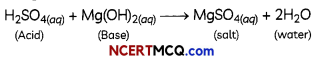Related Theory:
When an acid reacts with a base it forms salt and water. As a result, acidic properties disappear The process is called neutralisation. For a neutral solution. pH is 7. The solution having pH 7 wilL turn green in colour in universal indicator.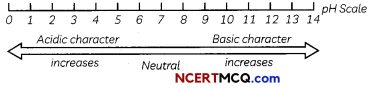This online pH calculator is designed to determine the pH of an aqueous solution of a given chemical compound.

Class 10 Science Chapter 2 MCQ Question 3.
Which of the following saLts does not contain water of crystatUsatlon?
(a) Blue vitriol
(b) Baking soda
(c) Washing soda
(d) Gypsum

Acid Base And Salts Class 10 MCQ Question 4.
A visually challenged student has to perform a lab test to detect the presence of acid in a given solution. The acid-base indicator preferred by him will be:
(a) Blue litmus
(b) Clove oil
(c) Red cabbage extract
(d) Hibiscus extract
(b) Clove oil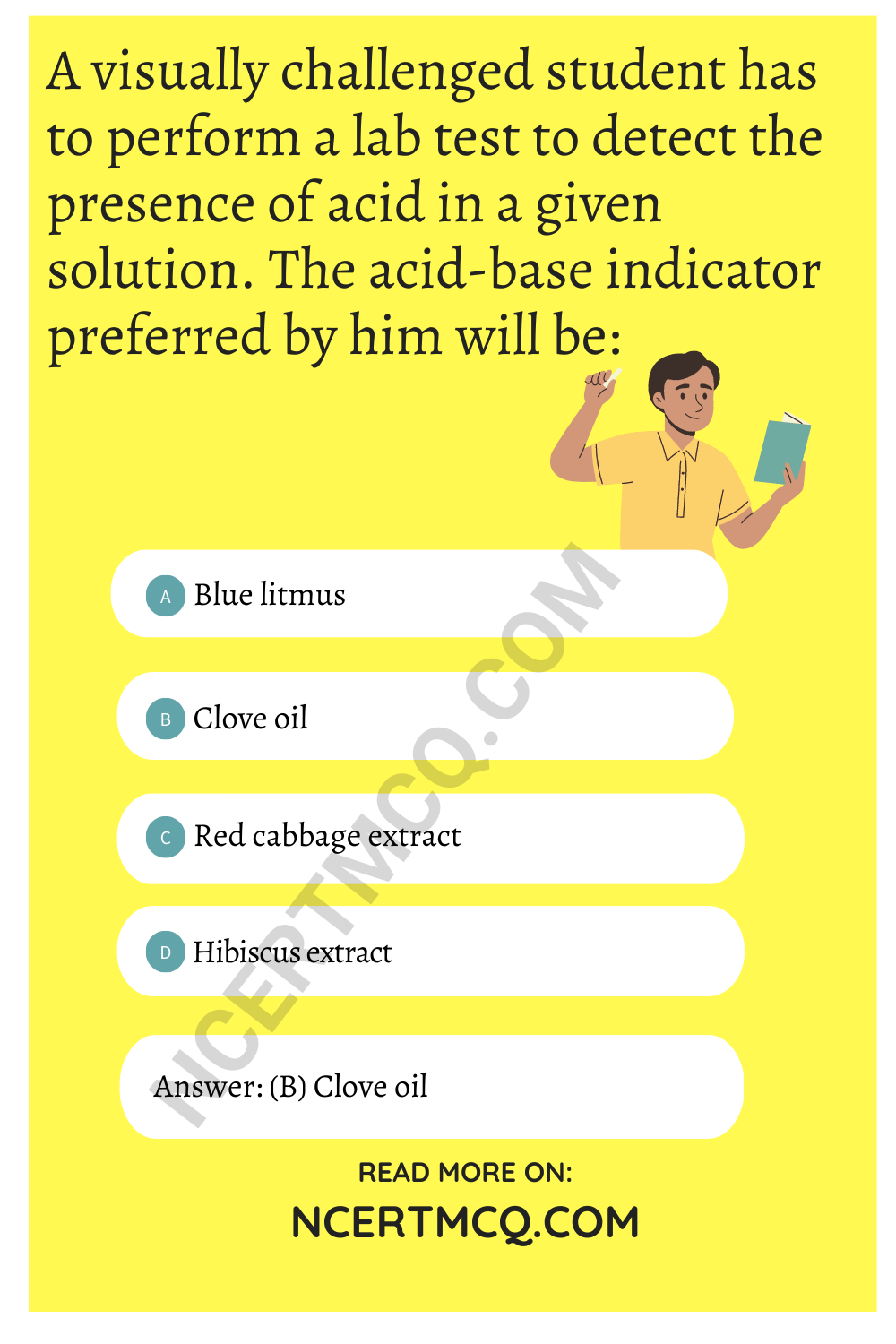Explanation: Clove oil is an olfactory indicator. These are the substances that give one type of odour in an acidic medium so a visually challenged student prefers to use clove oil as an acid-base indicator.

As in basic solutions, the smell of clove oil disappears while the smell is retained when mixed with an acid, on the other hand, blue litmus, red cabbage extract and hibiscus extract wouLd not be used as acid-base indicators because in these indicators there will be a change in the colour.

Class 10 Acids Bases And Salts MCQ Question 5.
Baking soda is a mixture of:
(a) Sodium carbonate and acetic acid
(b) Sodium carbonate and tartaric acid
(c) Sodium hydrogen carbonate and tartaric acid
(d) Sodium hydrogen carbonate and acetic acidMCQ Of Acid Base And Salts Class 10 Question 6.
Which of the following gives the correct increasing order of acid strength?
(a) Water < acetic acid < hydrochloric acid
(b) Water < hydrochloric acid < acetic acid
(c) Acetic acid < water < hydrochloric acid
(d) Hydrochloric acid < water < acetic acid

Ch 2 Science Class 10 MCQ Question 7.
What is observed when we pour a drop of acetic acid first on red and then on blue litmus papers?
(a) Red litmus paper becomes colourless and blue litmus paper remains blue.
(b) Red litmus paper turns blue and blue litmus paper remains blue.
(c) Red litmus paper remains red and blue litmus paper turns red.
(d) Red litmus paper turns blue and blue litmus paper turns red.

Class 10 Science Ch 2 MCQ Question 8.
Sodium hydrogen carbonate when added to acetic acid evolves a gas. Which of the following statements are true about the gas evolved?
(I) It turns lime water milky.
(II) It extinguishes a burning splinter.
(III) It dissolves in a solution of sodium hydroxide.
(IV) It has a pungent odour.
(a) (I) and (II)
(b) (I), (II) and (III)
(c) (II), (III) and (IV)
(d) (I) and (IV)
(b) (I), (II) and (III)

Explanation: Reaction of sodium hydrogen carbonate with acetic acid forms sodium acetate and water with carbon dioxide (CO2) gas.CO2 does not have a pungent smell but it shows all the other three properties:

1. turns lime water milky,
2. is a non-supporter of combustion and
3. absorbed by strong alkalis such as NaOH.

MCQ Questions For Class 10 Science Chemistry Chapter 2 Question 9.
Common salt besides being used in the kitchen can also be used as the raw material for making:
(I) Washing soda
(II) Bleaching powder
(III) Baking soda
(IV)Slaked Urne
(a) (I) and (II)
(b) (I), (II) and (IV)
(c) (I) and (III)
(d) (I), (III) and (IV)

Acids Bases And Salts MCQ Question 10.
To protect against tooth decay we are advised to brush our teeth regularly. The nature of the toothpaste commonly used is:
(a) Acidic
(b) Neutral
(c) Basic
(d) Corrosive
(c) Basic

Explanation: The toothpaste commonly used is alkaline or basic in nature as they contain mild bases such as sodium fluoride or sodium bicarbonate in their composition.

The base reacts with the acid formed during bacterial action in the mouth and neutralises its bad effects. Thus, preventing tooth decay. So, they can neutralize the effect of extra acids being formed in the mouth cavity which are mainly responsible for tooth decay.

Related Theory
When we eat sweet things, the pH of our mouth falls below 5.5 (moderately acidic) as the oral bacteria release acid while acting on sugars present in our food. The acidic conditions are capable of corroding the enamel which is made up of calcium phosphate. This causes the tooth to decay.MCQ Questions For Class 10 Science Chapter 2 Question 11.
Match the chemical substances given in Column I with their appropriate application given in Column II:

 Column I Column II (A) Bleaching Powder (1) Preparation of glass (B) Baking Soda (2) Production of H2 and Cl2 (C) Washing Soda (3) Decolourisation (D) Sodium Chloride (4) Antacid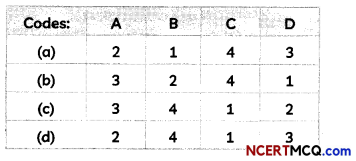Chapter 2 Science Class 10 MCQ Question 12.
Which of the following phenomena occur, when a small amount of acid is added to water?
(I) Ionisation
(II) Neutralisation
(III) Dilution
(IV)Salt formation
(a) (I) and (II)
(c) (II) and (III)
(b) (I) and (III)
(d) (II) and (IV)

Class 10 Chemistry Chapter 2 MCQ Question 10.
To protect against tooth decay we are advised to brush our teeth regularly. The nature of the toothpaste commonly used is:
(a) Acidic
(b) Neutral
(c) Basic
(d) Corrosive
(c) Basic

Explanation: The toothpaste commonly used is alkaline or basic in nature as they contain

Class 10 Acid Base And Salt MCQ Question 13.
A sample of soil is mixed with water and allowed to settle. The clear supernatant solution turns the pH paper yellowish-orange. Which of the following would change the colour of this pH paper to greenish-blue:
(a) Lemon juice
(b) Vinegar
(c) Common salt
(d) An antacid
(d) An antacid

Explanation: pH paper gives greenish-blue colour in a weak alkaline medium so antacid [Mg(OH)2] which is an alkaline compound will show greenish-blue color on pH paper.

Related Theory
Lemon fruit contains citric acid, vinegar has acetic acid and common salt is the neutral salt the clear super natant solution turns the pH paper yellowish-orange means the given sample of soil is acidic so it can be neutralised by base/alkaline solution.

MCQ On Acids Bases And Salts Class 10 Question 14.
Which of the following is not a mineral acid?
(a) Hydrochloric acid
(b) Citric acid
(c) Sulphuric acid
(d) Nitric acidClass 10 Chapter 2 Science MCQ Question 15.
Which of the following is/are true when HCl(g) is passed through water?
(I) It does not ionise in the solution as it is a covalent compound.
(II) It ionises in the solution.
(III) It gives both hydrogen and hydroxyl ions in the solution.
(IV) It forms hydronium ion in the solution due to the combination of hydrogen ion with water molecule.
(a) Only (I)
(b) Only (III)
(c) (II) and (IV)
(d) (III) and (IV)
(c) (II) and (IV)

Explanation: HCl, is a polar covalent compound, easily ionises in water to form hydronium (H3O+) and chloride ions (Cl). HCl (a strong acid) ionises completely in water to produce H+ and Cl ions. H+ ion combines with water molecules to produce hydronium ions.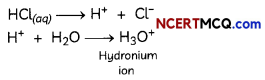Question 16.
Which among the following is not a base?
(a) NaOH
(b) KOH
(c) NH4OH
(d) C2H5OH
(d) C2HsOH
Explanation: C2H5OH is not a base. C2H5OH is an organic compound with -OH functional group that is known as alcohol. It cannot give OH ions in its solution. It cannot dissociate ions in a solution. Thus, it cannot be a basic compound.

Question 17.
Identify the correct representation of reaction occurring during the chloralkali process.
(a) 2NaCl(l) + 2H2O(l) → 2NaOH(l) + Cl2(g) + H2(g)
(b) 2NaCl(l) + 2H2O(aq) → 2NaOH(aq) + Cl2(g) + H2(aq)
(c) 2NaCl(aq) + 2H2O(l) → 2NaOH(aq) + Cl2(aq) + H2(aq)
(d) 2NaCl(aq) + 2H2O(l) → 2NaOH(aq) + Cl2(g) + H2(g)

Question 18.
Which of the following statements is true for acids?
(a) Bitter and change red litmus to blue.
(b) Sour and change red litmus to blue.
(c) Sour and change blue litmus to red.
(d) Bitter and change blue litmus to red.Question 19.
Zinc granules on treating with an acid X, form zinc sulphate (ZnSO4) salt along with the evolution of a gas Y, which burns with a pop sound when brought near to a burning candle. Identify acid X and gas evolved Y.
(a) X-sulphuric acid and Y-oxygen gas
(b) X-hydrochloric acid and Y-oxygen gas
(c) X-sulphuric acid and Y-hydrogen gas
(d) X-hydrochloric acid and Y-hydrogen gas [Diksha]
(c) X-sulphuric acid and Y-hydrogen gas

Explanation: When an acid reacts with a metal, hydrogen gas is liberated. In the given reaction, when dilute sulphuric acid reacts with zinc granules, hydrogen gas is liberated and zinc sulphate solution is formed:The presence of hydrogen gas is tested by bringing a lighted candle near it. When a lighted candle is brought near the test tube containing hydrogen gas, it burns with a ‘pop’ sound making a little explosion.

Hence, acid X is sulphuric acid and gas Y is hydrogen gas.

Question 20.
Which of the following solutions in water does not conduct electricity?
(a) Hydrochloric acid
(b) Sodium chloride
(c) Glucose
(d) Sulphuric acid [Diksha]
(c) Glucose

Explanation: The aqueous solution of an acid conducts electricity because of the presence of charged particles called ‘ions’ in it. When hydrochloric acid (HCl) is dissolved in water, its aqueous solution contains hydrogen ions (H+) and chloride ions (Cl). These ions carry electric currents. So, due to the presence of H+ and Cl ions, a solution of hydrochloric acid conducts electricity.

On the other hand, the hydrogen-containing compound such as glucose is not categorized as acid because it does not produce hydrogen ions or some other ions when dissolved in water and hence does not conduct electricity. Therefore, the answer is glucose.Question 21.
Which of the following will turn phenolphthalein pink?
(a) NaOH(aq)
(b) HCl(aq)
(c) CH3COOH(aq)
(d) H2O

Question 22.
Which one of the following is not a use of washing soda:
(a) Sodium carbonate (washing soda) is used in glass, soap and paper industries.
(b) It is used in the manufacture of sodium compounds such as borax.
(c) Sodium carbonate can be used as a clean¬ing agent for domestic purposes.
(d) It is used for disinfecting water.
(d) It is used for disinfecting water.

Explanation: The compound that is used for disinfecting water is bleaching powder. How¬ever, washing soda is used for removing the permanent hardness of water.

Question 23.
The approximate pH values of four salts is given below. Select the row(s) containing the correct information.

 Name of Salt PH (I) Potassium Sulphate 10 (II) Ammonium nitrate 5 (III) Sodium acetate 3 (IV) Sodium hydrogen carbonate 8

(a) Both (I) and (II)
(b) Both (II) and (III)
(c) Both (III) and (IV)
(d) Both (II) and (IV)

Question 24.
Zinc granules on treating with a substance X, form a salt sodium zincate along with the evolution of a gas Y which burns with a pop sound when brought near a burning candle. Identify the substance X and gas evolved Y.

 X Y (a) Acetic acid Hydrogen (b) Sodium hydroxide Hydrogen (c) Sodium hydroxide Oxygen (d) Zinc hydroxide Hydrogen

(b) X is Sodium hydroxide and Y is hydrogen.

Explanation: When zinc granules react with sodium hydroxide, salt sodium zincate is formed along with hydrogen gas, which burns with a pop sound.

The equation of the reaction taking place is:
2NaOH(aq) + Zn(s) → Na2ZnO2(aq) + H2(g)Question 25.
Which of the following salts belong to the same family of salts?
(I) sodium chloride and sodium acetate
(II) calcium sulphate, magnesium sulphate
(III) sodium carbonate and sodium hydrogen -carbonate
(IV) sodium chloride and magnesium sulphate
(a) Both (I) and (II)
(b) Both (I) and (III)
(c) (I), (II) and (III)
(d) (I), (III) and (IV)

Question 26.
A student noted his observations regarding the acidic or basic nature of salts as below:
(I) Sodium chloride is a neutral salt
(II) Ammonium chloride is a basic salt
(III) Sodium carbonate is a neutral salt
(IV) Copper sulphate is an acidic salt Select the incorrect observations:
(a) Both (I) and (III)
(b) Both (II) and (III)
(c) Both (I) and (IV)
(d) Both (II) and (IV)
(b) Both (II) and (III)

Explanation: The salts of strong acids and strong bases give neutral solutions. Therefore, sodium chloride is a neutral salt as it is obtained from sodium hydroxide (a strong base) and hydrochloric acid (a strong acid).

The salts of strong acids and weak bases give acidic solutions. Therefore, ammonium chloride and copper sulphate are acidic salts as ammonium chloride is obtained from ammonium hydroxide (a weak base) and hydrochloric acid (a strong acid), and copper sulphate is obtained from copper hydroxide (a weak base) and sulphuric acid (a strong acid).

The salts of weak acids and strong bases give basic solutions. Therefore, sodium carbonate is a basic salt as it is obtained from carbonic acid (a weak acid) and sodium hydroxide(a strong base).

### Assertion Reasoning questions Class 10 Science Chapter 2

For the following questions, two statements are given – one labelled Assertion (A) and the other labeled Reason (R). Select the correct answer to these questions from the codes (a), (b), (c) and (d) as given below:
(a) Both (A) and (R) are true and (R) is the correct explanation of the (A).
(b) Both (A) and (R) are true, but (R) is not the correct explanation of the (A).
(c) (A) is true, but (R) is false.
(d) (A) is false, but (R) is true.

Question 27.
Assertion,(A): HCl gas does not change the colour of dry blue litmus paper.
Reason (R): Acids always produce hydrogen ions.
(b) Both (A) and (R) are true, but (R) is not the correct explanation of the (A).

Explanation: HCl gas does not change the colour of dry litmus paper but changes colour of moist litmus paper as acids produce hydrogen ions only in solution.Question 28.
Assertion (A): Generally, the colour of indicators changes in a particular pH range.
Reason (R): Indicators are weak acids or weak base and exhibit different colours in molecular form and ionic form.
(a) (A) and (R) are true and (R) is the correct explanation of the (A).

Explanation: Since indicators are weak acids or weak bases, their percentage of existence in molecular state and in ionized state depends on the strength of acidic/basic/neutral solution to which they are added. Hence their colours change at a particular pH range.

Question 29.
Assertion (A): Zinc reacts with sodium hydroxide solution and hydrogen gas is evolved.
Reason (R): All metals react with bases to evolve hydrogen gas.

Question 30.
Assertion (A): While diluting an acid, water is slowly added to acid with constant stirring.
Reason (R): The process of dissolving an acid in water is a highly exothermic reaction.

Question 31.
Assertion (A): Metal oxides are acidic in nature.
Reason (R): Calcium hydroxide reacts with carbon dioxide to form a salt and water.
(d) (A) is false, but (R) is true.

Explanation: The reaction of calcium hydroxide with carbon dioxide to form a salt and water is similar to the reaction between an acid and a base. Therefore, non-metal oxides are acidic in nature.Question 32.
Assertion (A): When copper sulphate crystals are heated in a dry boiling tube, they turn white.
Reason (R): Water of crystallization is the number of water molecules present in one formula unit of a salt.
(b) Both (A) and (R) are true but (R) is not the correct explanation of (A).

Explanation: Copper sulphate crystals that seem to be dry contain water of crystallisation. When we heat the crystals, this water is removed and the salt turns white.
CUSO4.5H2O → CuSO4 + 5H2O

Question 33.
Assertion (A): When copper oxide is added to dilute hydrochloric acid, the colour of the solution becomes blue-green.
Reason (R): Copper (II) chloride is formed.
(a) (A) and (R) are true and (R) is the correct explanation of the (A).

Explanation: When copper oxide is added to dilute hydrochloric acid, the colour of the solution becomes blue-green due to the formation of Copper (II) chloride, which is blue-green in colour.

(Competency Based Questions (CBQs))

Question 1.
A chemistry student pLaced a strip of blue litmus paper and a strip of red litmus paper in a glass dish. Then she added a drop of dilute sulphuric acid to each strip of litmus paper. She observed that the blue litmus paper turned red, but the red litmus paper did not change color. Next, she placed a drop of sodium hydroxide (NaOH) on other strips of blue and red litmus paper. This time, the red litmus paper turned blue, but the blue litmus paper did not change. Finally, she put a drop of distilled water on strips of blue and red litmus paper. Neither strip changed color. She repeated the tests several times with the same results. The student concluded that acids turn blue litmus paper red; bases, such as sodium hydroxide, turn red litmus paper blue. As water did not affect either red or blue litmus paper, she reasoned that water was not an acid or a base, but a neutral substance.

Keeping these results in mind, the student poured a little sodium hydroxide into a beaker containing red and blue litmus paper. Then she added hydrochloric acid (HCl) drop by drop until the solution became neutral. She determined that a new, neutral substance had formed in the beaker. The substance was table salt, or sodium chloride (NaCl), which is one of many salts formed from an acid and a base.
(A) If a drop of an unknown substance turns blue litmus paper red, but does not change red litmus paper, what will be the substance – an acid or a base?

(B) When strips of blue and red litmus paper are put in a beaker filled with a clear solution, neither litmus paper changes colour. What can you say about the solution?
The solution must be neutral.

(C) Based on the results of this experiment, when would salt be formed?

(D) What happens when nitric acid is added to egg shell?
Calcium carbonate is main component of egg shell so when we add nitric acid to egg shell, it reacts with calcium carbonate to form soluble calcium nitrate and water with brisk effervescence of carbon dioxide gas.
CaCO3(s) + 2HNO3(aq) → Ca(NO3)2(aq) + CO2(g) + H2O

Question 2.
When we smile, our teeth become visible. So, we should ensure that we have a beautiful set of teeth as it makes our smile even more beautiful! Tooth enamel is the thin outer covering of the tooth. This tough shell is the hardest tissue in the human body. Enamel covers the crown which is the part of the tooth that’s visible outside of the gums.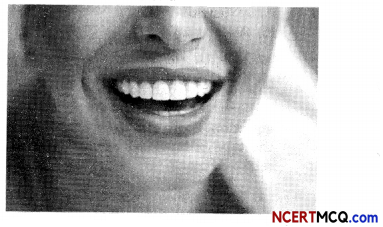Calcium phosphate is present in tooth enamel. Its nature is:
(a) Basic
(b) Acidic
(c) Neutral
(d) Amphoteric
(a) Basic

Explanation: Calcium phosphate Ca3(PO4)2 is a basic salt, as it is formed by the combination of a weak acid (phosphoric acid) and a slightly stronger base (calcium hydroxide).Question 3.
Patrick read about an industrial process for the electrolysis of sodium chloride solutions.
It is the technology used to produce chlorine and sodium hydroxide which are commodity chemicals required by industry. 35 million tons of chlorine were prepared by this process in 1987. Industrial-scale production began in 1892. Usually, the process is conducted on a brine (an aqueous solution of NaCl), in which case NaOH, hydrogen, and chlorine are formed. When using calcium chloride or potassium chloride, the products contain calcium or potassium instead of sodium. Related processes are known that use molten NaCl to give chlorine and sodium metal or condensed hydrogen chloride to give hydrogen and chlorine.
(A) Name the process referred to in the above passage.
The process referred to in the passage is chlor-alkali process because of the products formed – chlor for chlorine and alkali for sodium hydroxide.

(B) Where are the products of the above process produced?

(C) Write the chemical equation for the reaction taking place in the process.
The chemical equation for the reaction taking place is:
2NaCl(aq) + 2H2O → 2NaOH(aq) + Cl2(g) + H2(g)

(D) How is bleaching powder prepared from one of the products formed in this industrial process?

Question 4.
Neetu’s friend suffered a fracture in her foot while riding her bicycle. She had to be admitted to the nursing home and the doctor plastered her foot.The chemical formula for Plaster of Paris is:
(a) CaSO4.2H2O
(b) CaSO4.H2O
(c) CaSO4.$$\frac{1}{2}$$H2O
(d) 2CaSO4.H2O
(c) CaSO4. $$\frac{1}{2}$$ H2O

Explanation: Chemical name of Plaster of Paris is calcium sulphate hemihydrate. It is written in this form because two formula units of CaSO4 share one molecule of water.

Related Theory
When gypsum is heated at 373K, it loses water molecules.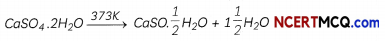It is a white powder used by doctors for supporting fractured bones. It is also used for making toys, materials for decoration and for making surfaces smooth.

Question 5.
Sabina studied in history about Mahatma Gandhi’s Dandi March and came to know that sodium chloride was an important symbol in our struggle for freedom. She started exploring from the internet as she was anxious to know what all we can do with this salt other than using it as a table salt to make food tasty. Table salt is also used to preserve food, facilitates transport of nutrients and oxygen and is used as raw material to form various salts.

Based on the understanding of the given passage and the related studied concepts, answer the following questions:
(A) Name two salts of daily use for which common salt (sodium chloride) is the raw material.
(B) Draw a diagram of chloralkali process and write its equation.Question 6.
While performing experiments in chemistry lab, one should always wear a laboratory coat and be very careful while performing experiments. Some students have the bad habit of creating nuisance for other students which distracts the students and may cause serious accidents such as spilling of acid or can cause burn injuries.If a few drops of concentrated acid accidentally spills over the hand of a student, what should be done?
(a) Wash the hand with a saline solution
(b) Wash the hand immediately with plenty of water and apply a paste of sodium hydrogen carbonate
(c) After washing with plenty of water, apply a solution of sodium hydroxide on the hand
(d) Neutralise the acid with a strong alkali
(b) Wash the hand immediately with plenty of water and apply a paste of sodium hydrogen carbonate

Explanation: In such an accident, washing the hands with water will dilute the acid and wash it out. Any remaining acid will be neutralized with sodium hydrogen carbonate (baking soda) as it is a base. This will minimize the effect of damage caused by acid to the skin.

Remember, too strong a base (like NaOH) can be corrosive.

Question 7.
Take about 2 mL of dilute NaOH solution in a test tube and a small amount of copper oxide in a beaker. Add two drops of phenolphthalein solution in the test tube. Add dilute HCl solution in both the test tube and the beaker drop by drop while stirring. Now add a few drops of NaOH to the mixture in the test tube.
(A) When two drops of phenolphthalein solution are added to the test tube,
(a) The solution turns pink in colour
(b) The solution turns colourless
(c) The solution turns red
(d) The solution turns yellow
(a) The solution turns pink in colour

Explanation: Phenolphthalein is a colourless reagent which is used as an indicator as it changes the colour to pink in a basic solution. As dilute NaOH is a basic solution, its colour changes to pink on adding a few drops of phenolphthalein solution.

(B) @Four students recorded their observations on adding dilute HCL to the beaker containing copper oxide. Select the correct observation:

 Student Observation-I Observation-II (a) Solution becomes colourless Copper oxide dissolves (b) Solution becomes blue-green in colour Copper oxide dissolves (c) Solution becomes blue green in colour Copper oxide is formed as a precipitate (d) Solution becomes colourless Copper chloride solution is colourless

(C) Select the correct observation(s):
When dilute HCl solution is added to the test tube drop by drop (after adding two drops of phenolphthalein solution) and then a few drops of NaOH solution are added to this mixture:
(I) The solution turns coLourless on adding dilute HCl
(II) The solution turns pink on adding dilute HCl
(III) The solution turns colourless again on adding NaOH to the mixture.
(IV) The solution turns pink again on adding NaOH to the mixture
(a) Both (I) and (III)
(b) Both (II) and (III)
(c) Both (I) and (IV)
(d) Both (II) and (IV)

(D) Identify the option that correctly describes the products formed in the test tube and beaker on adding dilute HCl:

 Test Tube Beaker (a) Sodium chloride + hydrogen Copper chloride + hydrogen (b) Sodium chloride + water Copper chloride + hydrogen (C) Sodium chloride + hydrogen Copper chloride + water (d) Sodium chloride + water Copper chloride + water

(d) Test Tube: Sodium chloride + water; Beaker: Copper chloride + water

Explanation: The products formed in the test is sodium chloride and water and that in beaker is copper chloride and water.

When sodium hydroxide reacts with dilute hydrochloric acid, it forms sodium chloride and water:
When copper oxide reacts with HCl, it forms copper chloride and water:
CuO(s) + 2HCl(aq) → CuCl2(aq) + H2O(l)

(E) Select the incorrect statement(s):
(I) Reaction of sodium hydroxide with dilute hydrochloric acid is a displacement reaction.
(II) Reaction of copper oxide with dilute hydrochloric acid is a double displacement reaction.
(III) Reaction of sodium hydroxide with dilute hydrochloric acid is a neutralization reaction.
(IV) Reaction of copper oxide with dilute hydrochloric acid is a neutralization reaction.
(a) Only (I)
(b) Only (II)
(c) Both (I) and (IV)
(d) Both (II) and (III)
(a) Only (I)

Explanation: Reaction of sodium hydroxide with dilute hydrochloric acid is a neutralization reaction which is also a double displacement reaction as products are formed by the exchange of ions between the reactants.

Similarly, the reaction of copper oxide with dilute hydrochloric acid is also a neutralization reaction which is also a double displacement reaction.

Whereas in a displacement reaction, a more reactive substance displaces a less reactive substance from its compound as in the reaction between a metal such as zinc and dilute hydrochloric acid.Question 8.
Sumit along with his friend went to a nearby shopping mall. They were standing under a tree when an ant stung his friend on his hands due to which his friend’s hand was painting a lot.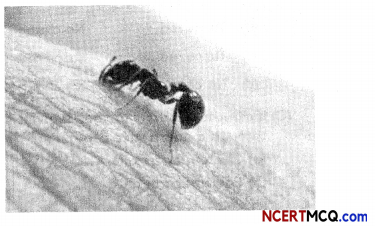Name the acid along with its chemical formula present in ant sting.
The acid present in ant sting is methanoic acid (formic acid). The chemical formula is HCOOH. Explanation: When an ant stings, it leaves formic acid (Methanoic acid) which causes pain and irritation. To get relief from the sting, mild base like baking soda can be applied on the stung.

Question 9.
Take about 1g solid NaCl in a clean and dry test tube and set up the apparatus as shown in Figure.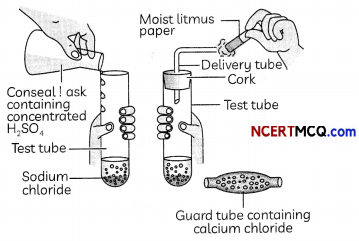Add some concentrated sulphuric acid to the test tube.
(A) The gas produced in the test tube is:
(a) Hydrogen
(b) Hydrogen chloride
(c) Hydrogen sulphide
(d) Sulphur dioxide
(b) Hydrogen chioride
Explanation: When concentrated sulphuric acid is added to sodium chloride, the following reaction takes place:

2NaCl+ H2SO4→ Na2SO4 + 2HCl

(B) A student tested the gas coming out of the delivery tube first with dry blue litmus paper and then with wet litmus paper and noted down the following observations. Select the correct observation:

 Dry Blue Litmus Paper Wet Blue Litmus Paper (a) No change Turns red (b) Turns red No change (c) Turns red Turns red (d) No change No change

(C) If climate is humid, the gas evolved is passed through a guard tube containing:
(a) Calcium hydroxide
(b) Calcium carbonate
(c) Calcium sulphate
(d) Calcium chloride
(d) Calcium chloride

Explanation: During the preparation of hydrogen chloride gas, the gas is usually passed through the guard tube containing calcium chloride during humid climate, as calcium chloride is a good drying agent and absorbs moisture from the gas.

(D) Select the incorrect statement(s):
(I) Hydrogen ions in HCl are produced even in absence of water.
(II) Hydrogen ions can exist alone.
(III) Hydrogen ions exist after combining with water molecules.
(IV) Hydrogen ions in HCl are produced only in presence of water
(a) Only (I)
(b) Both (I) and (II)
(c) Both (I) and (III)
(d) Both (II) and (IV)
(b) Both (I) and (II)
Explanation: The separation of H+ ions from HCl molecules cannot occur in the absence of water.
HCl +H2O → H3O+ + Cl
Hydrogen ions cannot exist alone, but they exist after combining with water molecules. Thus hydrogen ions must always be shown as H+(aq) or hydronium ion (H30+).
H+ + H2O → H3O+

(E) Hydrogen ions must always be shown as H+(aq) or hydronium ion (H30+) as:
(a) Hydrogen ions combine with water to form hydronium ions
(b) Hydrogen gas dissociates into ions.
(c) All acids dissociate into hydrogen ions in presence of a base.
(d) Hydrogen ions separate from acids on passing electricity.

Question 10.
Acids and bases represent two important classes of chemical compounds. In every day life, acids and bases play a role in everything from the digestion of the foods you eat to the function of the medicine you take and even the cleaning products you use. Without acids and bases, many of the products in your home today would not have much use. Acids and bases are also important in atmospheric, geological, and physiological processes because they have unique chemical properties. Acids and bases have unique chemical properties because of the atomic composition of these compounds and how these compounds interact with other atoms and molecules.
The pH of some common substances is shown below:
(A) The nature of calcium phosphate present in tooth enamel is:
(a) Basic
(b) Amphoteric
(c) Acidic
(d) Neutral

(B) What is the pH value of saliva after meal?
(a) between 0 and 4
(b) between 4 and 5
(c) between 5 and 6
(d) between 6 and 7
(c) between 5 and 6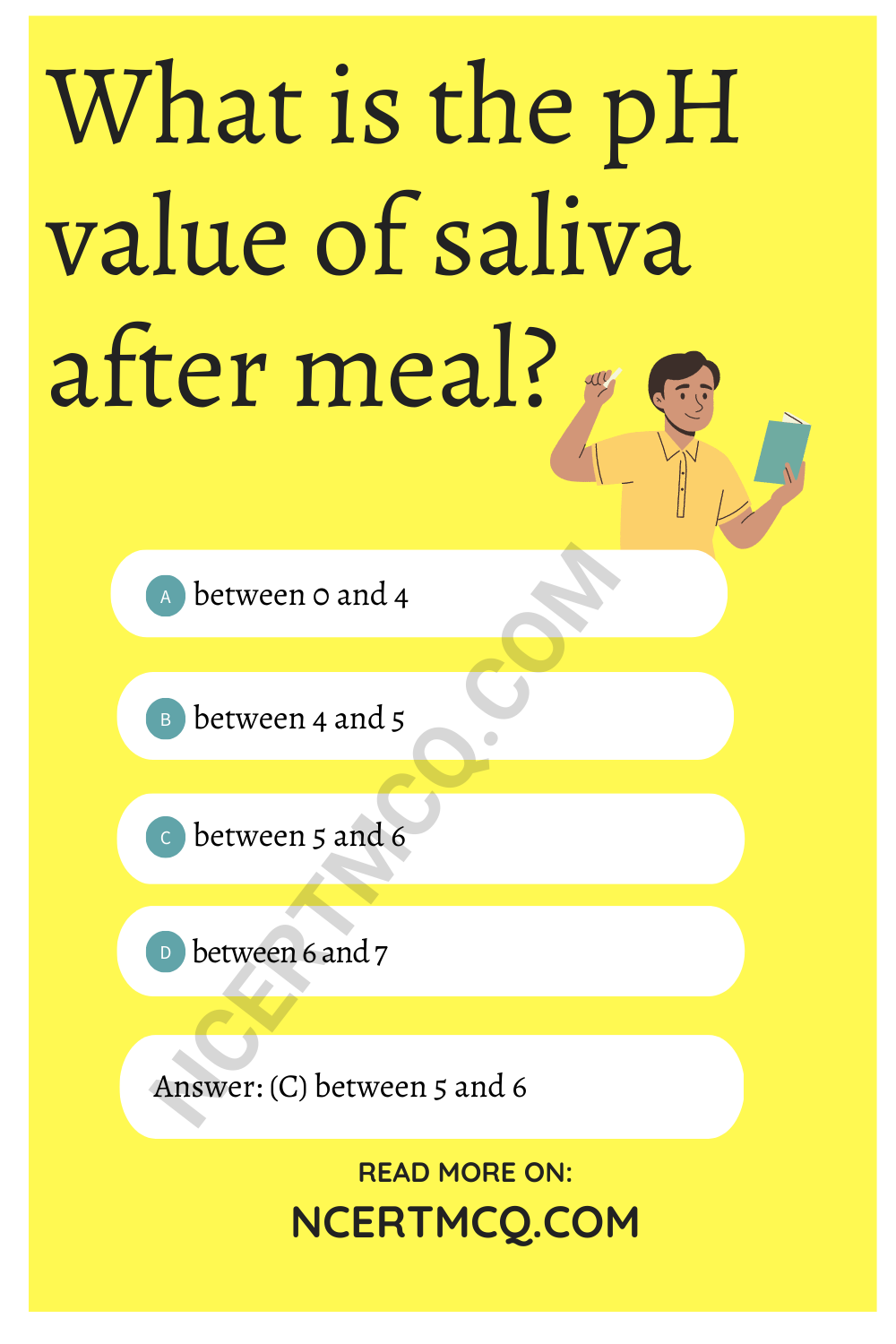Explanation: The pH of saliva after meals is around 5.8 due to the increased activity of bacteria on the food that we eat.

(C) Given below are four statements. Select the incorrect statement(s):
(I) Pain and irritation during indigestion is due to the excess acid produced by the stomach.
(II) People use antacids to get rid of this pain which are bases.
(III) Milk of magnesia is an antacid as it is a mild acid.
(IV) Antacids neutralize the excess acid produced in the stomach.
(a) Only (I)
(b) Only (II)
(c) Only (III)
(d) (III) and (IV)
(c) Only (III)

Explanation: Antacids are bases which help in getting rid of the pain and irritation during indigestion by neutralizing the excess acid produced in the stomach. Milk of magnesia or magnesium hydroxide is a mild base that is used as an antacid.

(D) The pH level of the human body is:
(a) Less than 7, acidic
(b) Equal to 7, Neutral
(c) More than 7, basic
(d) None of the above

(E) A student tabulated the naturally occurring acidic substances and the acid contained in them.

 Natural Source Acid found (I) Vinegar Methanoic acid (II) Nettle sting Acetic acid (III) Tomato Oxalic acid (IV) Orange Citric acid

Select the option containing the correct acid found in them:
(a) Both (I) and (II)
(b) Both (III) and (IV)
(c) Both (II) and (IV)
(d) Both (I) and (III)
(b) Both (III) and (IV)

Explanation: Vinegar contains acetic acid, whereas nettle sting contains methanoic acid.Question 11.
If you think the best way to put out a fire is by dousing it with water, you’d be surprised to know you’re wrong. As it turns out, not all fires are created equal, and there is more than one way to extinguish them safely. There are situations where you should not use water to deal with a fire at all. For example, an electrical fire.Name the chemicals used in acid fire extinguishers and the gas that evolved from it when it is used?
The chemicals used in soda acid fire extinguishers are sodium hydrogen carbonate and sulphuric acid.
The gas evolved when the two reactants react is carbon dioxide which is used for extinguishing electrical fires.
The reaction taking place between the two chemicals is
2NaHCO3 + H2SO4 → Na2SO4 + 2CO2 + 2H2O

Question 12.
The reaction between MnO2 with HCl is depicted in the following diagram. It was observed that a gas with bleaching abilities was released.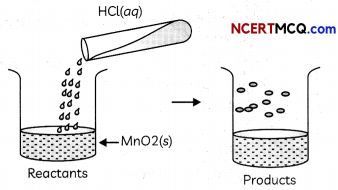(A) The chemical reaction between MnO2 and HCl is an example of:
(a) displacement reaction
(b) combination reaction
(c) redox reaction
(d) decomposition reaction.
(c) redox reaction

Explanation: When MnO2 reacts with HCl, the following reaction takes place in which chlorine gas is also evolved, which has bleaching properties.
MnO2 + 4HCl → MnCl2 + Cl2 + 2H2O

In this reaction, Hydrochloric acid is oxidized to chlorine and Manganese dioxide is reduced to manganese dichloride. As both oxidation and reduction are taking place in this reaction, it is a redox reaction.

(B) Chlorine gas reacts with ……………… to form bleaching powder.
(a) dry Ca(OH)2
(b) dil. solution of Ca(OH)2
(c) cone, solution of Ca(OH)2
(d) dry CaO
(a) dry Ca(OH)2
Explanation: Bleaching powder is produced by the action of chlorine on dry slaked lime [Ca(OH)2],
Ca(OH)2 + Cl2 → CaOCl2 + H2O

(C) Identify the correct statement from the following:
(a) MnO2 is getting reduced whereas HCl is getting oxidized
(b) MnO2 is getting oxidized whereas HCl is getting reduced.
(c) MnO2 and HCl both are getting reduced.
(d) MnO2 and HCl both are getting oxidized.
(a) Mn02 is getting reduced whereas HCl is getting oxidized.
Explanation: In the reaction between
MnO2 and HCl, MnO2 is getting reduced to manganese dichloride as it is losing oxygen atoms. Whereas, HCl is getting oxidized to chlorine by the removal of oxygen.

Related Theory
Oxidation is the addition of oxygen to a substance or the removal of hydrogen from a substance.
Reduction is the addition of hydrogen to a substance or removal of oxygen from a substance.
The oxidising agent is the substance that provides oxygen for oxidation or which removes hydrogen.
Reducing agent is the substance which provides hydrogen for reduction or which removes oxygen.

(D) In the above-discussed reaction, what is the nature of MnO2?
(a) Acidic oxide
(b) Basic oxide
(c) Neutral oxide
(d) Amphoteric oxide
(b) Basic oxide
Explanation: As MnO2 reacts with HCl to form salt, water and chlorine gas, it is a basic oxide since it reacts with an acid. Moreover, oxides of metals are basic in nature whereas oxides of non-metals are acidic in nature.

(E) What will happen if we take dry HCl gas instead of aqueous solution of HCl?
(a) Reaction will occur faster.
(b) Reaction will not occur.
(c) Reaction rate will be slow
(d) Reaction rate will remain the same.
(b) Reaction wiil not occur

Explanation: A substance shows its acidic properties only in presence of water as it is able to dissociate into H+ ions, which is responsible for its acidic properties.

So, if we use dry HCl, then no reaction will take place as HCl will not be able to dissociate into ion\$ as shown by the following reaction:
HCl + H2O H3O+ + ClQuestion 13.
Frothing in Yamuna:
The primary reason behind the formation of the toxic foam is high phosphate content in the wastewater because of detergents used in dyeing industries, dhobi ghats and households. Yamuna’s pollution level is so bad that parts of it have been labelled ‘dead’ as there is no oxygen in it for aquatic life to survive.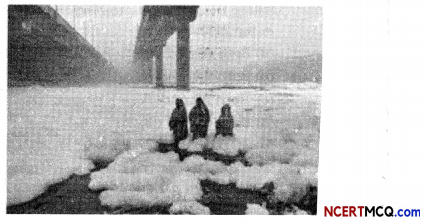(A) Predict the pH value of the water of river Yamuna if the reason for froth is high content of detergents dissolved in it.
(a) 10-11
(b) 5-7
(c) 2-5
(d) 7
(a) 10-11

Explanation: Detergents are basic in nature having pH value of 10-11. As the froth has a high content of detergents dissolved in it, the pH value of water of river Yamuna will be 10 -11.

(B) Which of the following statements is correct for the water with detergents dissolved in it?
(a) low concentration of hydroxide ion (OH)and high concentration of hydronium ion (H3O+)
(b) high concentration of hydroxide ion (OH) and low concentration of hydronium ion (H3O+)
(c) high concentration of hydroxide ion (OH) as well as hydronium ion (H3O+)
(d) equal concentration of both hydroxide ion (OH) and hydronium ion (H3O+).
(b) high concentration of hydroxide ion (OH) and low concentration of hydronium ion (H3O+)

Explanation: As detergents are basic in nature, the concentration of hydroxide ions will be high and the concentration of hydronium ions will be less.

Related Theory
When bases are dissolved in water, they dissociate into hydroxide ions.
For example, NaOH(aq) → Na+(aq) + CH(aq)
KOH(aq) → K+(aq) + OH(aq)

(C) The table provides the pH value of four solutions P, Q, R and S

 Solution PH Value P 2 Q 9 R 5 S 11

Which of the following correctly represents the solutions in increasing order of their hydronium ion concentration?
(a) P > Q > R> S
(b) P > S > Q > R
(c) S < Q < R< P
(d) S < P < Q < R
(c) S < Q < R. < P

Explanation: The pH of a substance is related to the concentration of hydrogen or hydronium ions. An acid has a high concentration of hydronium ions and a low pH value whereas a base has a high pH value and a low concentration of hydronium ions.

So, we can say, lower the pH value, the more is the concentration of hydronium ions. Therefore, the hydronium ion concentration is least in S, followed by Q, R and then P which has the maximum concentration of hydronium ions.

(D) High content of phosphate ion in river Yamuna may lead to:
(a) decreased level of dissolved oxygen and increased growth of algae
(b) decreased level of dissolved oxygen and no effect of the growth of algae
(c) increased level of dissolved oxygen and increased growth of algae
(d) decreased level of dissolved oxygen and decreased growth of algae
(a) decreased level of dissolved oxygen and increased growth of algae

Explanation: When the amount of phosphate ions in water increases, it leads to decreased level of dissolved oxygen which is harmful for the aquatic organisms. This leads to an increased growth of algae due to presence of nutrients.

Related Theory
Eutrophication is the process in which a water body becomes overly enriched with nutrients, leading to plentiful growth of simple plant life. The excessive growth (or bloom) of algae and plankton in a water body are indicators of this process.

(E) If a sample of water containing detergents is provided to you, which of the following methods will you adopt to neutralize it?
(a) Treating the water with baking soda
(b) Treating the water with vinegar
(c) Treating the water with caustic soda
(d) Treating the water with washing soda
(b) Treating the water with vinegar

Explanation: As detergents are basic in nature, an acid will neutralize it. Out of the substances mentioned, only vinegar is acidic, as it is 5 – 8% solution of ethanoic acid in water.

Question 1.
What types of ions are formed: (a) when an acid is dissolved in water (b) when a base is dissolved in water?

Question 2.
‘A’ is a soluble acidic oxide and ‘B’ is a soluble base. Compared to pH of pure water, what will be the pH of (a) solution of A (b) solution of B?
pH of pure water is 7. As A is a soluble acid, its pH will be less than that of pure water whereas pH of B will be more than that of pure water as it is a soluble base.Question 3.
When electricity is passed through a common salt solution, sodium hydroxide is produced along with the liberation of two gases “X” and “Y”. “X” burns with a pop sound whereas “Y” is used for disinfecting drinking water. Identify X and Y.
The reaction is chlor-alkali process. As X burns with a pop sound, X is hydrogen gas. Y is chlorine gas which is used for disinfecting drinking water.

Question 4.
Which bases are called alkalis? Give one example of alkali.
The bases that are soluble in water are called alkalies. One example of an alkali is sodium hydroxide, NaOH.

Question 5.
Name the acid and base that have com stituted the salt ammonium nitrate

Question 6.
Oxides of metals are basic while those of non-metals are acidle, Explain.
Oxides of metals are basic as they react with acids to produce salt and water.
CuO + 2HCl → CuCl2 + H2O
Oxides of non-metals are acidic as they react with bases to produce salt and water.
CO2 + Ca(OH)2 → CaCO3 + H2O

Question 7.
Write a balanced chemical eauatin for the renction between sodium carbonals and hydrochloric acid indicating the physical state of reactions and the products.
The balanced equation for the reaction between sodium carbonate and hydrochloric acid is given below:
Na2CO3(s) + 2HCl(aq) → 2NaCl(aq) + H2O(l) + CO2(g)

Question 8.
Give reasons for the following:
(A) Only one half of water molecule is shown in the formula of Plaster of Paris.
(B) Sodium hydrogen carbonates is used as an antacid.
(C) On strong heating, blue coloured copper sulphate crystals turn white.

Question 9.
A student prepared solutions of (I) an acid and (II) a base in two separate beakers. She forgot to label the solutions and litmus paper is not available in the laboratory. Since, both the solutions are colourless, how will she distinguish between the two?
In the absence of litmus, other natural or synthetic substances can be used to test acid and bases. Such substances are called indicators. Indicators such as methyl orange and phenolphthalein can be used to test the nature of a solution.

These indicators show change in their colour in acidic, neutral and basic solutions. We can also use natural indicators such as turmeric and grape juice. A few indicators with characteristic colour change are shown below:Question 10.
Fill in the missing data in the given table.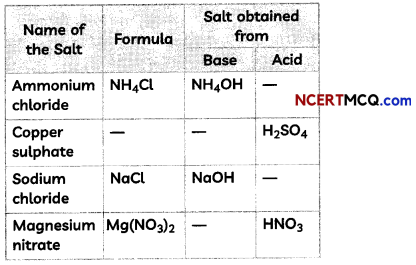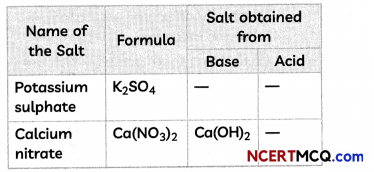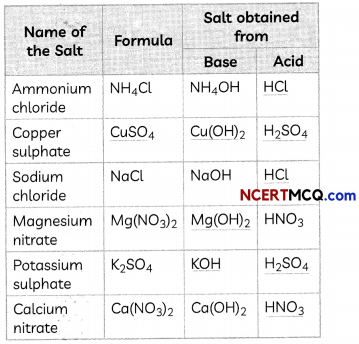1. Acid: HCl
NH4OH + HCl → NH4Cl + H2O

2. Formula: CuSO4 and base: Cu(OH)2
H2SO4 + Cu(OH)2 → CuSO4 + 2H2O

3. Acid: HCl
NaOH + HCl → NaCl + H2O

4. Base: Mg(OH)2
Mg(OH)2 + 2HNO3 → Mg(NO3)2 + 2H2O

5. Base: KOH and Acid: H2SO4
2KOH + H2SO4 → K2SO4 + 2H2O

6. Acid: HNO3
Ca(OH)2 + 2HNO3 → Ca(NO3)2 + 2H2O

Question 11.
How is washing soda prepared from sodium carbonate? Give its chemical equation. State the type of this salt. Name the type of hardness of water that can be removed by it?

Question 12.
(A) You are given two solutions A and B and their pH is 6 and 8 respectively. Answer the following:
(i) Which of the two solutions have more hydrogen ion concentration?
(ii) Which is acidic and which is basic?
pH of solution A = 6 pH of solution B = 8
(i) Solution A will have a higher hydrogen ion concentration. Explanation: We know that the pH of any solution is inversely proportional to the hydrogen ion concentration. This means that the solution that has lower pH number will have the higher hydrogen ion concentration.

(ii) Also solution A is acidic and solution B is basic because H+ ion concentration is higher in acidic solution.

(B) What is dilution?
Mixing an acid or base with water results in decrease in concentration of ions (H3O+/ OH) per unit volume. Such process is called dilution and the acid or base is said to be dituted. The process of dissolving an acid or a base in water is a highly exothermic reaction.Question 13.
Equal lengths of magnesium ribbons are taken in test tubes A and B. Hydrochloric acid (HCl) is added to test tube A, while acetic acid (CH3COOH) is added to test tube B. In which test tube will the fizzing occur more vigorously and why?

Question 14.
Crystals of a substance changes their color on heating in a closed vessel but regained it after sometime, when they were allowed to cool down.
(A) Name one such substance.
One such substance whose crystals changes its colour from blue to white on heating in a closed vessel for some time is hydrated copper sulphate, CuSO4.5H2O.

(B) Explaain the phenomenon involved.
Hydrated salts contain molecules of water known as water of crystallization. When such crystals are heated in a closed container, they lose their water of crystallization and hence change of colour is observed.

Related Theory:
When a salt which has lost its water of crystallization due to heating is left to cool down, it absorbs moisture from air and regains its water of crystallisation and thus, also its color.Question 15.
The pH of the mouth of a person is lower than 5.5. What changes will occur in EMB his mouth? How these changes can be controlled? Write any two measures.